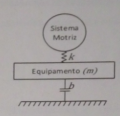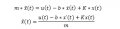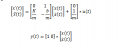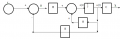# State Space model for mechanical system

#### Current_Source

Joined Dec 10, 2015
16
Hello!

I am trying to solve the following problems:

Consider a machine whose mass m is supported by a na anti-vibration support with an damping coefficient b. When in operation the drivetrain, of negligible mass, causes a displacement u on the rod, with an elastic coefficient K, according to the scheme shown in Figure.Taking as system output the displacement x of the equipment find a state space model for the system.
For this I gotTherefore I for the following matrixes:Is this correct?

Now I would like tocalculate a regulator with state feedback by placing the poles of the system in -1 and -2. Trace the respective block diagram.

Can anyone give an explanation on how can I calculate this? I was able to came up with the following diagramThanks